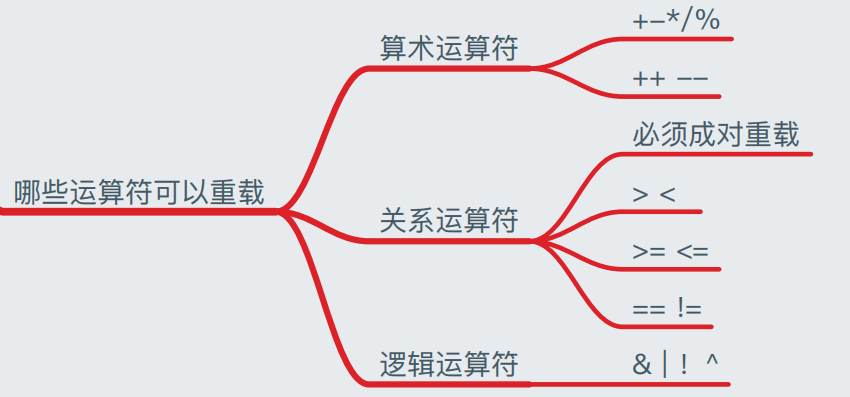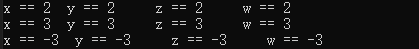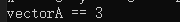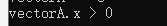2022-01-27 17:20:09

一些情况下，我们希望运算符能拥有一些我们我们自己定义的功能方便使用，这时候就会用到运算符重载。

以下代码比较简单地重载了加号，功能使两个box对象的length相加。

public static Box operator+ (Box b, Box c)
{
Box box = new Box();
box.length = b.length + c.length;
return box;
}

重载的运算符肯定是要被外部使用的，所以一般定义为public，它显然也是被类的所有对象共享的，所以用static修饰，重载的运算符和函数的功能是类似的，所以也有返回值类型和参数列表。

注意，条件运算符&&和||、赋值运算符=,+=, -=, *=, /=, %=都是不能被重载的。开发语言
更多相关内容
• 主要介绍了C#运算符重载用法,实例分析了C#中运算符重载的基本实现与使用技巧,需要的朋友可以参考下
• C# 运算符重载 ** 项目中把float,int封装了,在两个数据比较时候就忘了了这茬, 直接引用类型和引用类型比较,返回的指定为false, 当然可以获取数值进行比较,但不是一劳永逸的办法, 所以运算符重载就解决的问题. 在菜鸟...

**

## C# 运算符重载

**
项目中把float,int封装了,在两个数据比较时候就忘了了这茬,
直接引用类型和引用类型比较,返回的指定为false,
当然可以获取数值进行比较,但不是一劳永逸的办法,
所以运算符重载就解决的问题.
在菜鸟里看到了这篇文章,整理了一下,大家一起学习呀!

可以重定义或重载 C# 中内置的运算符。因此，程序员也可以使用用户自定义类型的运算符。重载运算符是具有特殊名称的函数，是通过关键字 operator 后跟运算符的符号来定义的。与其他函数一样，重载运算符有返回类型和参数列表。

可重载和不可重载运算符
下表描述了 C# 中运算符重载的能力：

运算符描述
+, -, !, ~, ++, –这些一元运算符只有一个操作数，且可以被重载。
+, -, *, /, %这些二元运算符带有两个操作数，且可以被重载。
==, !=, <, >, <=, >=这些比较运算符可以被重载。
&&, ll这些条件逻辑运算符不能被直接重载。
+=, -=, *=, /=, %=这些赋值运算符不能被重载。
=, ., ?:, ->, new, is, sizeof, typeof这些运算符不能被重载。
 public static bool operator == (Box lhs, Box rhs)
{
bool status = false;
if (lhs.value== rhs.value)
{
status = true;
}
return status;
}
public static bool operator !=(Box lhs, Box rhs)
{
bool status = false;
if (lhs.value!= rhs.value)
{
status = true;
}
return status;
}
public static bool operator <(Box lhs, Box rhs)
{
bool status = false;
if (lhs.value< rhs.value)
{
status = true;
}
return status;
}

public static bool operator >(Box lhs, Box rhs)
{
bool status = false;
if (lhs.value> rhs.value)
{
status = true;
}
return status;
}

public static bool operator <=(Box lhs, Box rhs)
{
bool status = false;
if (lhs.value<= rhs.value)
{
status = true;
}
return status;
}

public static bool operator >=(Box lhs, Box rhs)
{
bool status = false;
if (lhs.value>= rhs.value)
{
status = true;
}
return status;
}


operator 关键字用于在类或结构声明中声明运算符。运算符声明可以采用下列四种形式之一：

public static result-type operator unary-operator ( op-type operand )
public static result-type operator binary-operator ( op-type operand, op-type2 operand2 )
public static implicit operator conv-type-out ( conv-type-in operand )
public static explicit operator conv-type-out ( conv-type-in operand )


参数：

• result-type 运算符的结果类型。
• unary-operator 下列运算符之一：+ - ! ~ ++ — true false
• op-type 第一个（或唯一一个）参数的类型。
-operand 第一个（或唯一一个）参数的名称。
• binary-operator 其中一个：+ - * / % & | ^ << >> == != > < >= <=
• op-type2 第二个参数的类型。
-operand2 第二个参数的名称。
• conv-type-out 类型转换运算符的目标类型。
• conv-type-in 类型转换运算符的输入类型。

注意：
前两种形式声明了用户定义的重载内置运算符的运算符。并非所有内置运算符都可以被重载（请参见可重载的运算符）。op-type 和 op-type2 中至少有一个必须是封闭类型（即运算符所属的类型，或理解为自定义的类型）。例如，这将防止重定义整数加法运算符。
后两种形式声明了转换运算符。conv-type-in 和 conv-type-out 中正好有一个必须是封闭类型（即，转换运算符只能从它的封闭类型转换为其他某个类型，或从其他某个类型转换为它的封闭类型）。
运算符只能采用值参数，不能采用 ref 或 out 参数。
C# 要求成对重载比较运算符。如果重载了==，则也必须重载!=，否则产生编译错误。同时，比较运算符必须返回bool类型的值，这是与其他算术运算符的根本区别。
C# 不允许重载=运算符，但如果重载例如+运算符，编译器会自动使用+运算符的重载来执行+=运算符的操作。
运算符重载的其实就是函数重载。首先通过指定的运算表达式调用对应的运算符函数，然后再将运算对象转化为运算符函数的实参，接着根据实参的类型来确定需要调用的函数的重载，这个过程是由编译器完成。
任何运算符声明的前面都可以有一个可选的属性（C# 编程指南）列表。

展开全文前端
• ## C#运算符重载

千次阅读 2019-11-18 14:58:04
运算符重载：通过特定的语法，是某些运算符可以具备特殊的功能。关键字operator，修饰符必须为public static。 【注意事项】 1、参数的数量一定要和这个运算符操作的数量匹配。 2、参数类型和返回值类型可以随意设置...

运算符重载：通过特定的语法，是某些运算符可以具备特殊的功能。关键字operator，修饰符必须为public static。
【注意事项】
1、参数的数量一定要和这个运算符操作的数量匹配。
2、参数类型和返回值类型可以随意设置，但要合情合理。
3、可以重载的运算符有：算术运算符（+、-、*、/、++、–）、关系运算符（>、<、>=、<=、==、!=）、逻辑运算符（&、|、!、^）、位运算符（~）。
4、关系运算符如果想重载，那么必须要成对的重载，例如：重载了>，则必须重载<。
5、不可被重载的运算符有：赋值运算符、逻辑运算符中的（&&、||）。

重写语法代码如下：

using System;

public  struct Point
{
public double x;
public double y;
public Point(double x,double y)
{
this.x = x;
this.y = y;
}
//运算符重载，使加号具有新的功能
//可以实现两个Point对象直接相加，得到一个新的点
public static Point operator +(Point p1,Point p2)
{
return new Point(p1.x + p2.x, p1.y + p2.y);
}
//参数类型可以不相同
public static Point operator + (Point p1,int a)
{
return new Point(p1.x + a, p1.y + a);
}
//*号是二元运算符，因此必须有两个参数
public static Point operator *(Point p1,Point p2)
{
return new Point(1, 1);
}
//-号的身份有两种：1是加减乘除的减号，2是负号。为前者时是二元运算符，为后者是一元运算符。
public static Point operator - (Point p1)
{
return new Point(1, 1);
}
//【重载--运算符】练习
public static Point operator --(Point p1)
{
return new Point(p1.x-1,p1.y-1);
}
}

public class Program
{
public static void Main(string[] args)
{
Point p1 = new Point(1, 2);
Point p2 = new Point(2, 3);
Point p = p1 + p2;
//【重载--运算符】练习
Point p3 = new Point(1, 3);
p3--;
Console.WriteLine(p3.x);
Console.WriteLine(p3.x);
Console.WriteLine(p3.y);
p3 = new Point(1, 3);
--p3;
Console.WriteLine(p3.x);
Console.WriteLine(p3.y);
}
}

展开全文• 您可以重定义或重载 C# 中内置的运算符。因此，程序员也可以使用用户自定义类型的运算符重载运算符是具有特殊名称的函数，是通过关键字 operator 后跟运算符的符号来定义的。与其他函数一样，重载运算符有返回类型...
• PS:本系列笔记将会记录我此次在北京学习Unity开发...Part 1 运算符重载 运算符重载 重载后的运算符能够实现原运算符不能实现的功能 运算符包括(+ - * /) (> < >= <= == !=) (& | ! ^) 注意事项 >...

PS:本系列笔记将会记录我此次在北京学习Unity开发的总体过程，方便后期写总结，笔记为日更。
笔记内容均为 自己理解，不保证每个都对。
C#笔记未按照难度排列

## Part 1 运算符重载

运算符重载
重载后的运算符能够实现原运算符不能实现的功能
运算符包括(+ - * /) (> < >= <= == !=) (& | ! ^)
注意事项 >< >= <= == !=必须成对出现重载格式为：

 访问权限 static 返回类型 operator 运算符（参数）


例如：重载+号

        /// <summary>
/// 重载运算符 +号        让Vector4能够加上一个数字
/// operator +     表示对运算符+号的重载
/// static是必须的，表示全局
/// Vector4表示返回类型
/// </summary>
/// <param name="leftValue">等号左边Vector4</param>
/// <param name="tmpInt">右边的数字</param>
/// <returns></returns>
public static Vector4 operator +(Vector4 leftValue, int tmpInt)     //重载 +号，让Vector4类能够直接加上数字
{
leftValue.x += tmpInt;
leftValue.y += tmpInt;
leftValue.z += tmpInt;
leftValue.w += tmpInt;

return leftValue;
}

public static Vector4 operator +(Vector4 leftValue, Vector4 rightValue)     //重载 +号，让Vector4类能够直接加上Vector4类修饰的变量
{
leftValue.x += rightValue.x;
leftValue.y += rightValue.y;
leftValue.z += rightValue.z;
leftValue.w += rightValue.w;

return leftValue;
}


测试代码为：

            Vector4 vectorA = new Vector4(1);       //(1, 1, 1, 1)
Vector4 vectorB = new Vector4(1);       //(1, 1, 1, 1)
Vector4 vectorC = new Vector4(-3);      //(-3, -3, -3, -3)

vectorA = vectorA + 1;      //(1 + 1, 1 + 1, 1 + 1, 1 + 1) 即（2， 2， 2， 2）
vectorA.Debug();

vectorA = vectorA + vectorB;        //（2 + 1， 2 + 1， 2 + 1， 2 + 1）即（3, 3, 3, 3）
vectorA.Debug();        //输出结果为3   3    3   3
vectorC.Debug();        //输出结果为-3  -3  -3  -3


输出结果为：其他运算符同理

注意：重载 > < >= <= == !=的时候运算符要成对出现
例如重载 ==：

        public static bool operator ==(Vector4 leftValue, int tmpInt)
{
bool flag = false;
if (leftValue.x == tmpInt && leftValue.y == tmpInt && leftValue.z == tmpInt && leftValue.w == tmpInt)        //只要x y z w有一项不等于tmpInt即为false
flag = true;
return flag;
}

public static bool operator !=(Vector4 leftValue, int tmpInt)
{
bool flag = false;
if (leftValue.x != tmpInt && leftValue.y != tmpInt && leftValue.z != tmpInt && leftValue.w != tmpInt)        //只要x y z w有一项等于tmpInt即为false
flag = true;
return flag;
}


测试代码为：

            if (vectorA == 3)
{
Console.WriteLine("vectorA == 3");
}
else
{
Console.WriteLine("vectorA != 3");
}


输出结果为：重载布尔变量：

        public static bool operator true(Vector4 leftValue)
{
return leftValue.x > 0;
}

public static bool operator false(Vector4 leftValue)
{
return leftValue.x <= 0;
}


测试代码为：

            if (vectorA)
{
Console.WriteLine("vectorA.x > 0");
}
else
{
Console.WriteLine("vectorA <= 3");
}


输出结果为：展开全文Unity 笔记
• 下面是 Vector 的定义， 包含只读属性， 构造函数和重写的 ToString() 方法， 最后是运算重载符 struct Vector { public Vector(double x, double y, double z) { X = x; Y = y; Z = z; } public Vector...
• C#运算符重载----operator运算符重载的实现可重载和不可重载运算符实例原文链接 您可以重定义或重载**C#**中内置的运算符。因此，程序员也可以使用用户自定义类型的运算符。重载运算符是具有特殊名称的函数，是通过...
• 运算符重载的关键是在对象上不能总是只调用方法或属性， 有时还需要做一些其他工作，例如对数值进行相加，相乘或逻辑（比较对象）等。 假定已经定义了一个表示数学矩阵类。 在数学领域中，矩阵可以相加和相乘， 就...
• 可以重定义或重载 C# 中内置的运算符。因此，程序员也可以使用用户自定义类型的运算符重载运算符是具有特殊名称的函数，是通过关键字 operator 后跟运算符的符号来定义的。与其他函数一样，重载运算符有返回类型和...学习 开发语言
• C#运算符重载 您可以重定义或重载 C# 中内置的运算符。因此，程序员也可以使用用户自定义类型的运算符。重载运算符是具有特殊名称的函数，是通过关键字operator后跟运算符的符号来定义的。与其他函数一样，重载...java css 编程语言...

# c#运算符重载c# 订阅